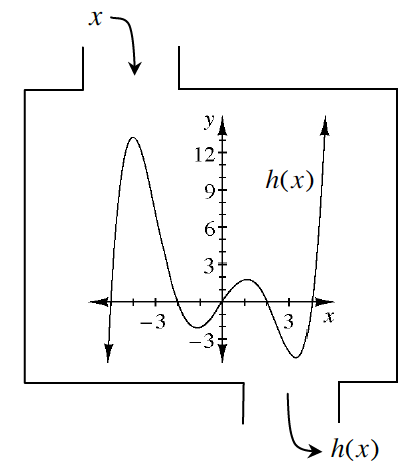### Home > GB8I > Chapter 2 Unit 2 > Lesson INT1: 2.1.1 > Problem2-8

2-8.

Examine the function $h(x)$ defined at right. Then estimate the values below.

1. $h(1)$

2. $h(3)$

3. $x$ when $h(x) = 0$

4. $h(−1)$

5. $h(−4)$

Look at the graph to estimate the $y$-value for each of the given $x$-values.

There are $5$ $x$-intercepts on the graph.

For part (c), you are being asked to find the values for $x$ when $y = 0$. (Find the $x$-intercepts).

$2$
$−4$